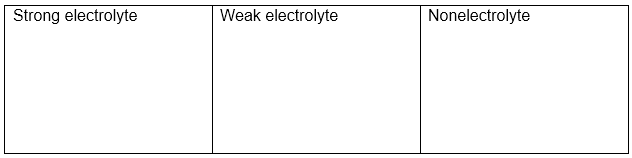# Problem: Each of the following reactions shows a solute dissolved in water. Classify each solute as a strong electrolyte, a weak electrolyte, or a nonelectrolyte.1. C(l) → C(aq)2. AB(aq) ⇌ A + (aq) + B − (aq)3. MN(aq) → M + (aq) + N − (aq)4. XZ(s) → X + (aq) + Z − (aq)5. P(s) → P(aq)Drag the appropriate items to their respective bins.If a solute produces ions when dissolved, it is called an electrolyte because the resulting ions in solution will allow the solution to conduct electricity. A solute that completely dissociates into ions is a stronger electrolyte than one that only partially dissociates into ions. If a solute remains as a molecule when dissolved, it is called a nonelectrolyte.In this tutorial, you will practice identifying substances as strong electrolytes, weak electrolytes, or nonelectrolytes.

###### FREE Expert Solution
94% (254 ratings)View Complete Written Solution
###### Problem Details

Each of the following reactions shows a solute dissolved in water. Classify each solute as a strong electrolyte, a weak electrolyte, or a nonelectrolyte.
1. C(l) → C(aq)
2. AB(aq) ⇌ A + (aq) + B − (aq)
3. MN(aq) → M + (aq) + N (aq)
4. XZ(s) → X + (aq) + Z (aq)
5. P(s) → P(aq)

Drag the appropriate items to their respective bins.If a solute produces ions when dissolved, it is called an electrolyte because the resulting ions in solution will allow the solution to conduct electricity. A solute that completely dissociates into ions is a stronger electrolyte than one that only partially dissociates into ions. If a solute remains as a molecule when dissolved, it is called a nonelectrolyte.

In this tutorial, you will practice identifying substances as strong electrolytes, weak electrolytes, or nonelectrolytes.